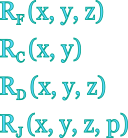## Carlson's Elliptic Integrals

This tool evaluates the Carlson's symmetric form of elliptic integrals: RF(x,y,z), RC(x,y), RD(x,y,z) and RJ(x,y,z,p). Arguments x, y, z should be generally non-negative, but more restrictions apply. Argument p should be non-zero. Enter the arguments below.

 x = y = z = p = Result: RF (x,y,z) = RC (x,y) = RD (x,y,z) = RJ (x,y,z,p) =